# Revealing Rates Through Partitive Division

Build fluency and flexibility with composed unit ratios and rates revealed through partitive division with these Math Is Visual Prompts.

## In This Set of Math Visual Prompts…

Students will explore equivalent ratios and reveal rates through partitive division.

## Intentionality…

This set of visual math talk prompts is taken from the Math Talk section of Day 2 in the Make Math Moments Problem Based Unit called Planting Flowers. The purpose of the Math Talk is to reinforce key concepts and big ideas from the first day of this problem based math unit including:

• There are two types of ratios; composed unit and multiplicative comparison;
• A composed unit is often (not always) a ratio with two distinct units;
• A composed unit can be scaled in tandem;
• Equivalent ratios are derived from the same rate;
• When you divide a composed unit through partitive division, you reveal a rate.

This set of visual math talk prompts, we will explicitly emerge two strategies for revealing a rate from a ratio: through partitive division via fair sharing and through scaling in tandem on a double number line.

## Preparing to Facilitate

Present the following composed unit ratios one at a time. Create a context to define the units for each ratio. Focus on ratios with two distinct units at this time. Ask students to determine and justify one or more equivalent ratios for each composed unit. Equivalent ratios can be revealed by scaling the composed unit up or down. For each ratio, reveal the rate by leveraging partitive division.

8 : 4

14 : 2

27 : 3

32 : 8

6 : 4

As you’ll notice with this set of animated Math Visual Prompts, we begin by introducing a composed unit ratio of 8 apples to 4 baskets which provides an extremely low floor for students to access and enter the problem.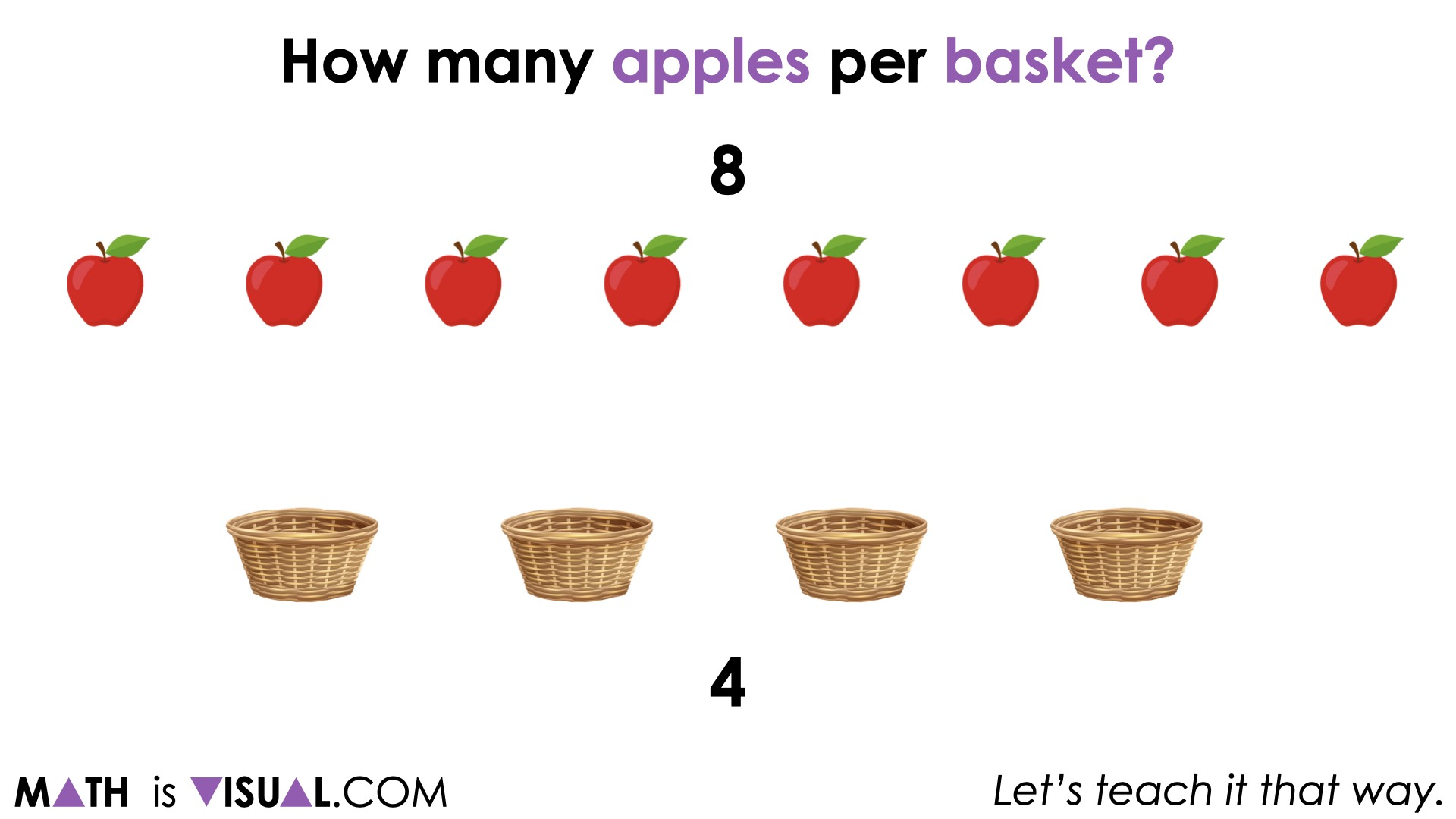It will almost seem obvious to many students that the 8:4 ratio they are given of apples to baskets is equivalent to 2:1.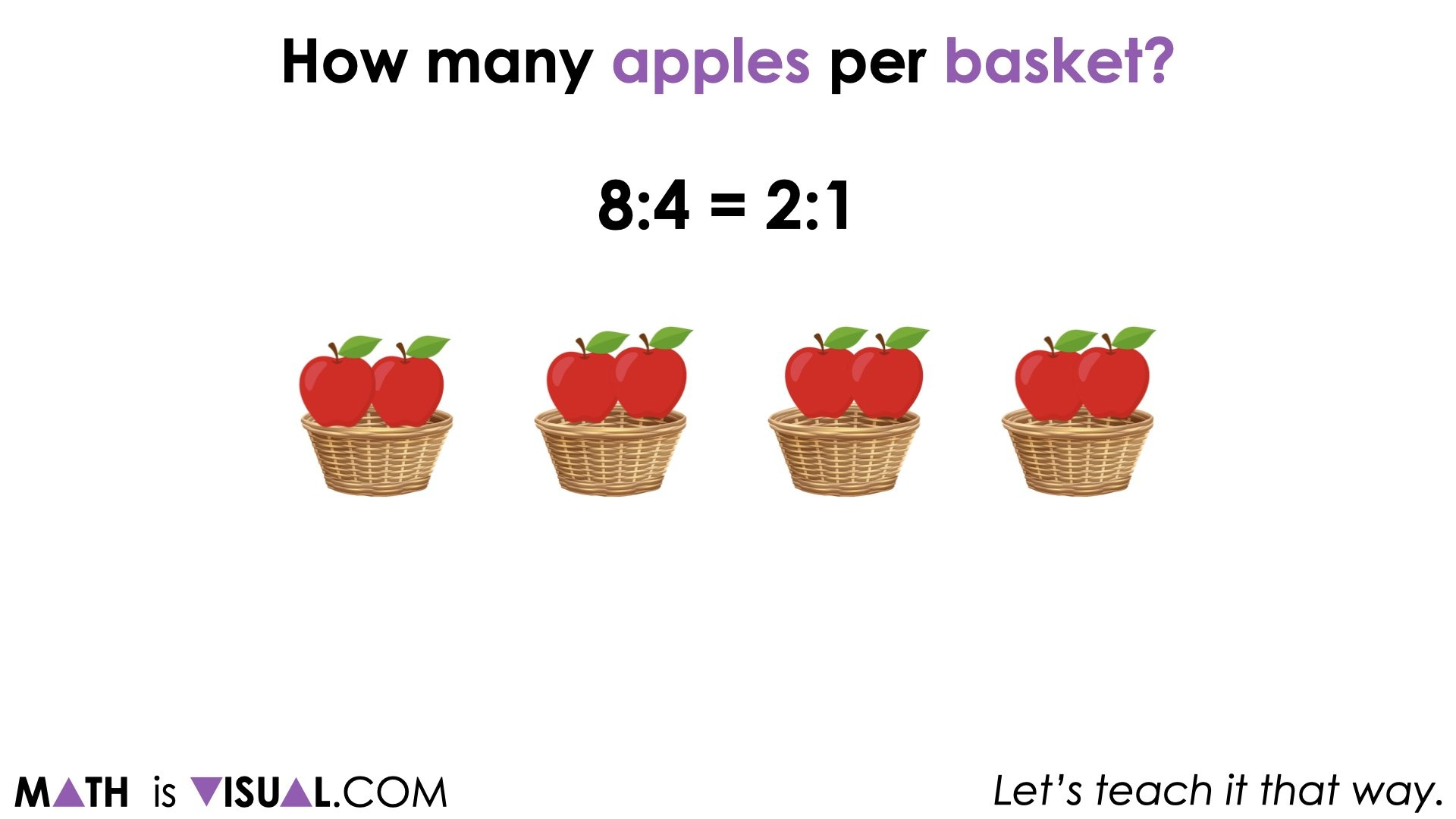What students (and many educators) may not realize, is that while we have scaled this composed unit ratio from 8:4 down to 2:1 by “fourthing” the ratio, we can also quickly reveal a rate of 2 apples per basket through partitive division.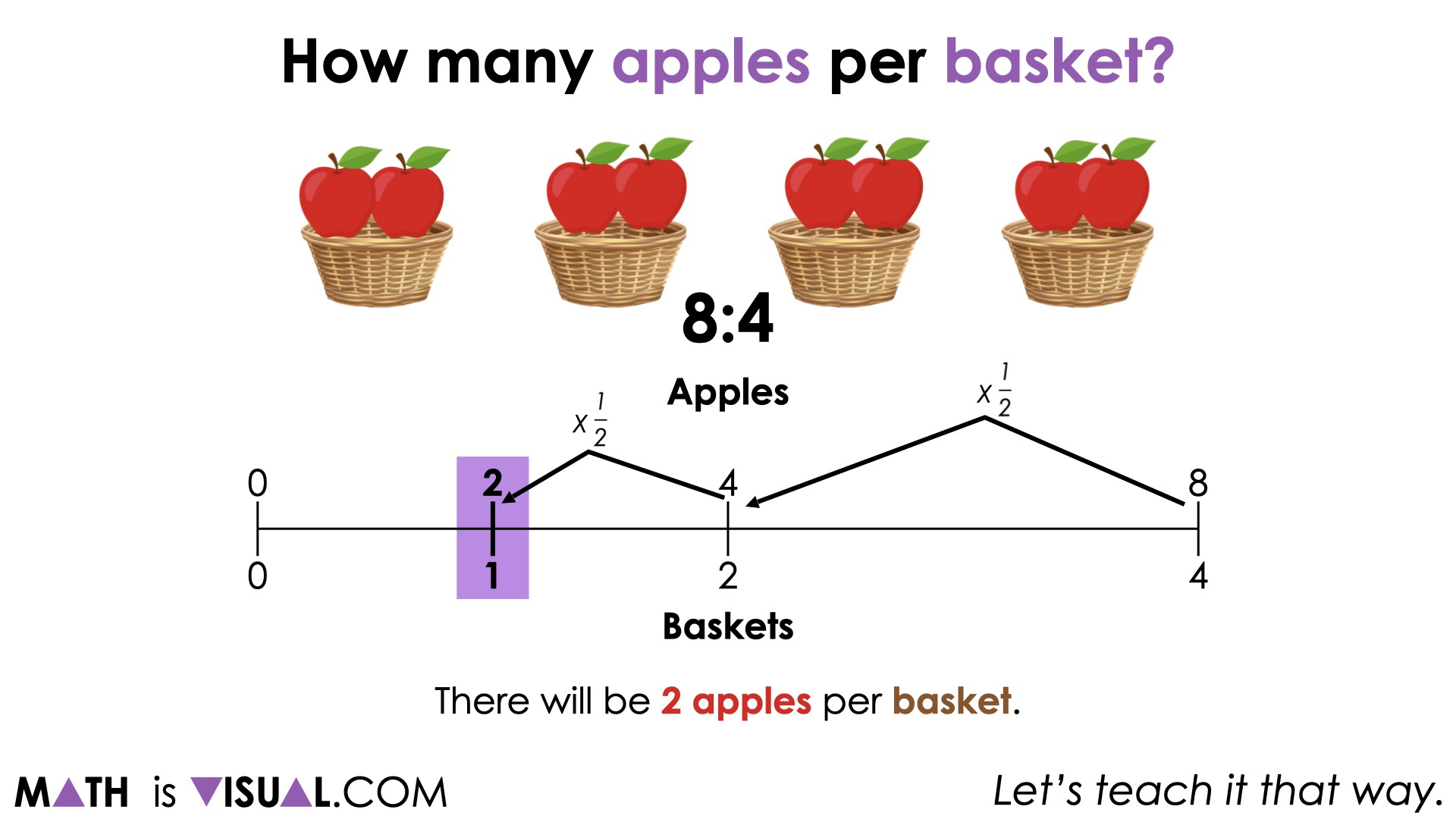The goal here is to give students an opportunity to work with ratios using their intuition. Also we aim to help them realize that while we can scale any ratio up or down to find an infinite number of equivalent ratios, scaling to a unit ratio provides access to reveal a rate through partitive division (without really having to divide at all).

We also emerge the double number line as a tool for thinking as well as a tool to represent thinking

Although there are five (5) ratios to work through in this animated Math Visual Prompt sets where we use partitive division via fair sharing (distributing apples equally to each basket) as well as through scaling in tandem on the double number line, be sure to dive into the final ratio where we reveal a fractional rate of apples per basket.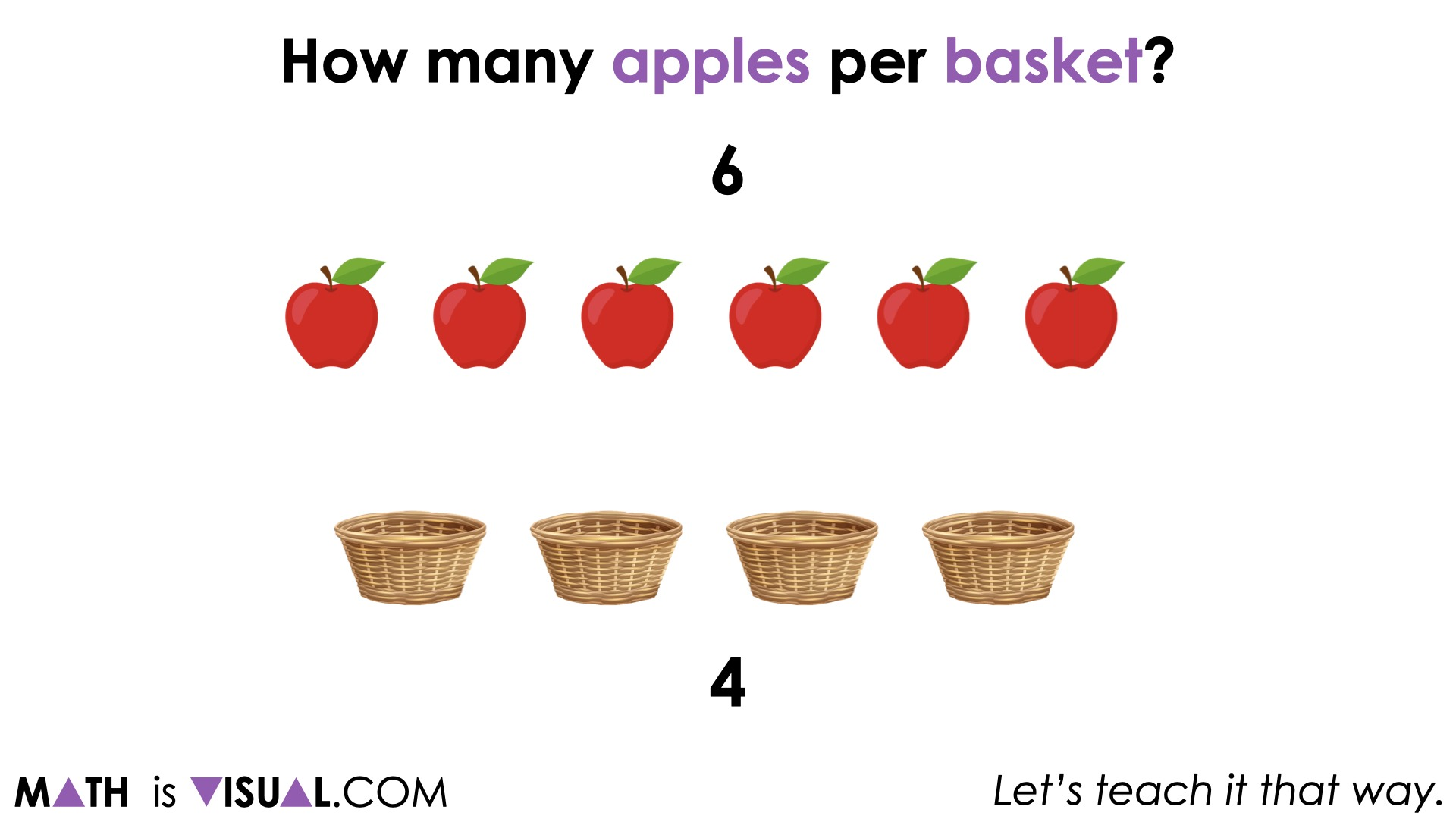Through partitive division and the fair sharing strategy, you can quickly see that each basket will receive 1 and 1 half apples, revealing a rate of 1 ½ apples per basket: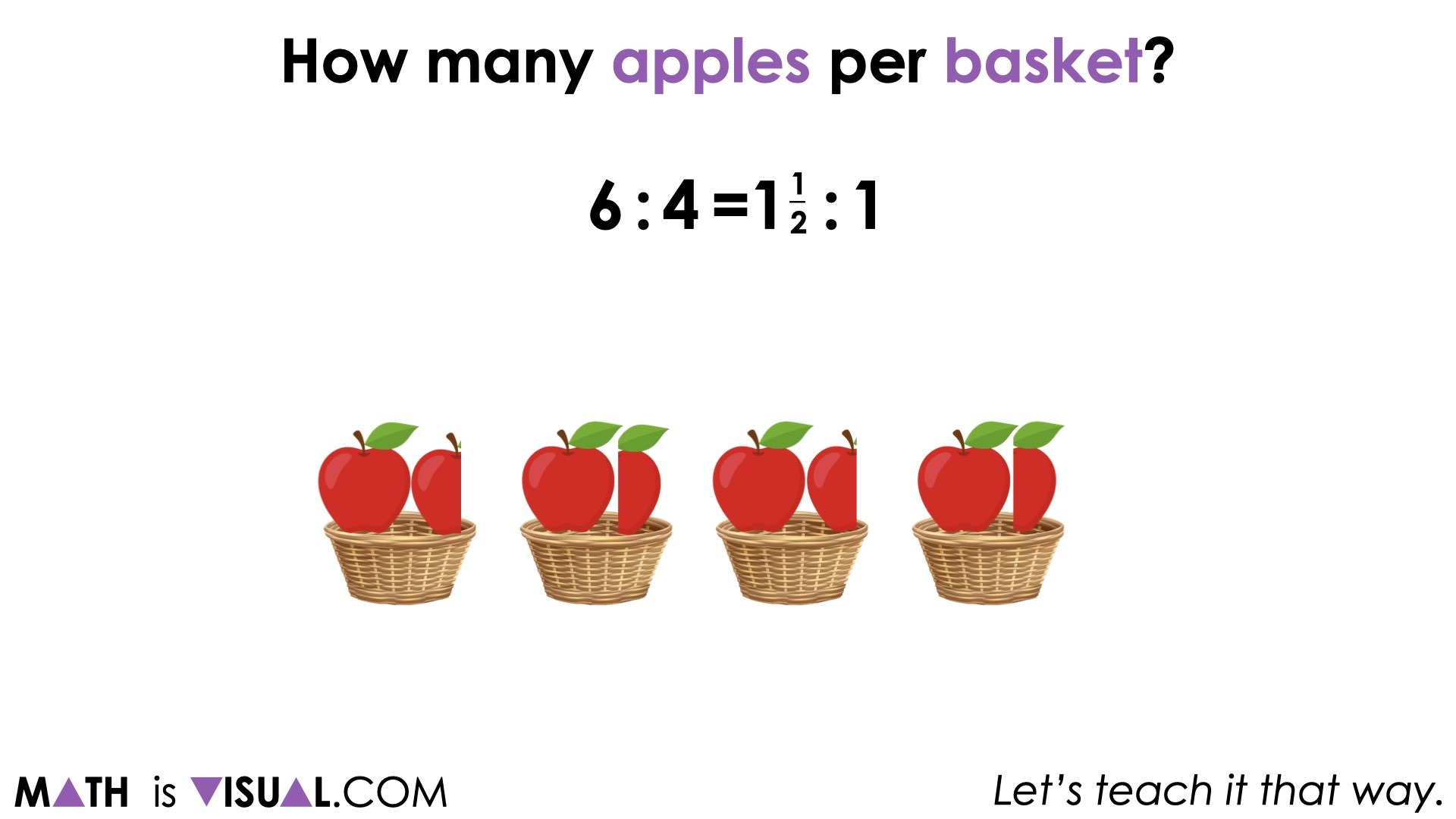Scaling in tandem by halving twice (or “fourthing”) reveals an equivalent ratio of 1.5:1 apples to baskets: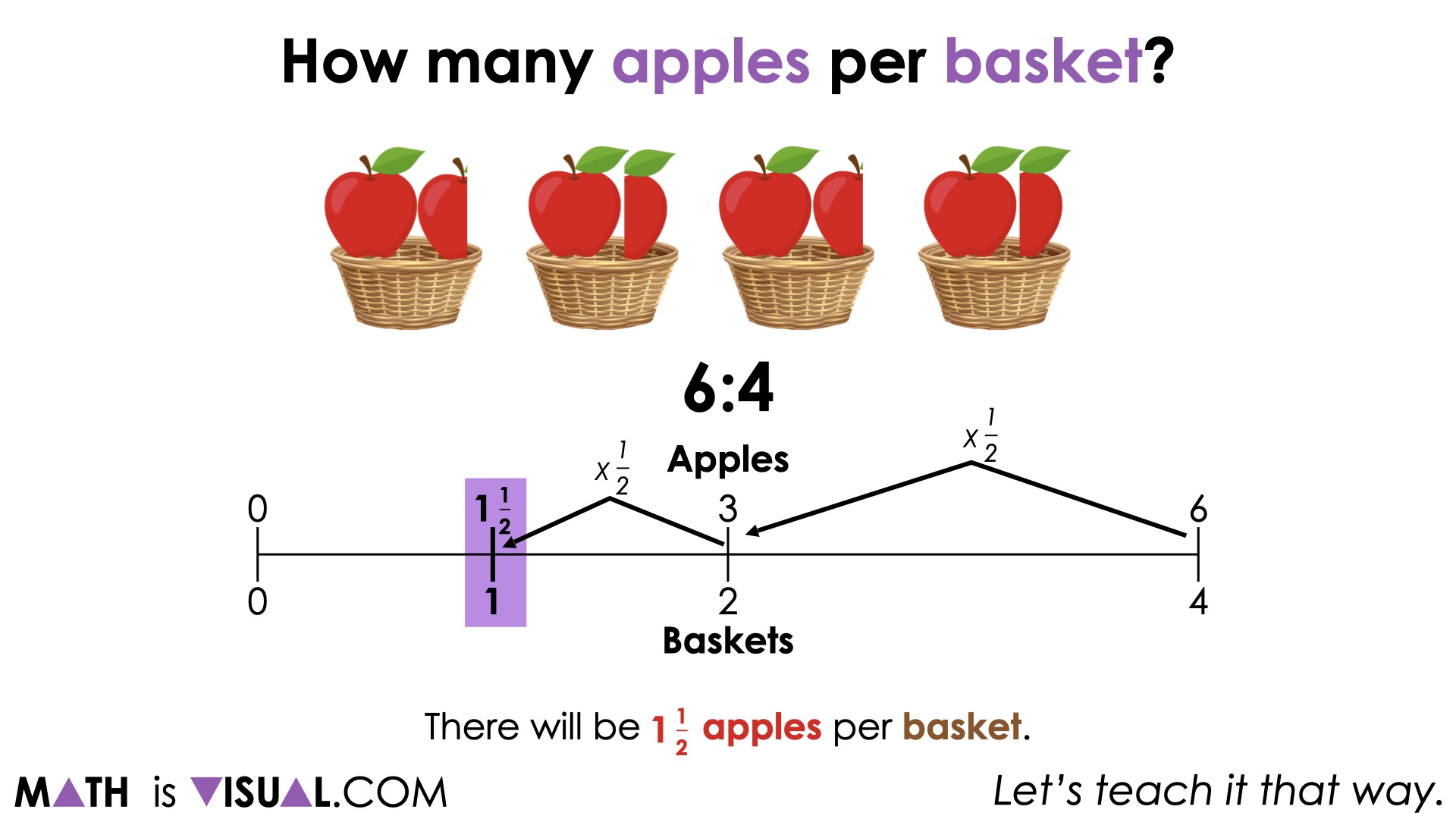Note that the model you are intentionally emerging from this set of Math Visual Prompts is a double bar model and/or a double number line.

Facilitator Note:

From each of these ratios, two rates can be derived. For example 8 apples : 4 baskets, the two rates can be revealed through partitive division:

and

## While Students Are Working…

Pay close attention to the strategies that students are using.

Are students:

• Using concrete materials to fair share?
• Using a concrete or visual array?
• Partitioning or scaling a bar model?
• Using a double number line?
• Using a ratio table?
• Counting on?
• Multiplicative thinking?

Document this thinking and use this data to help you determine which students you might consider strategically pulling individually or in small groups to offer guided instruction in order to move them along their developmental continuum.

## Want to Explore These Concepts & Skills Further?

The full 5-day unit of ratios and rates real world lessons can be accessed on the Make Math Moments Problem Based Units page.

Did you use this in your classroom or at home? How’d it go? Post in the comments!

Math IS Visual. Let’s teach it that way.